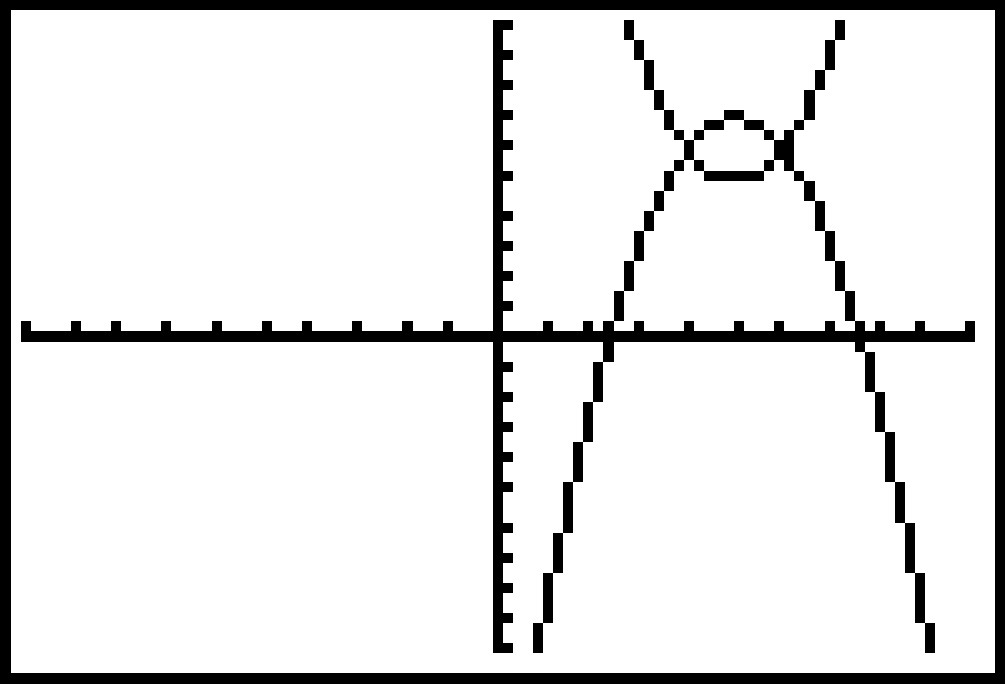# Knowledge Base

## Solution 11767: Graphing an Ellipse on a Texas Instruments Graphing Calculator.

### How do I graph an ellipse on a Texas Instruments graphing calculator?

To input an ellipse into the Y= Editor of a TI graphing calculator, the equation for the ellipse would need to solved in terms of y. The example below will demonstrate how to graph an ellipse.

For Example:
Graph an ellipse where a=1, b=1, and the center of the ellipse is at point (5,6).

1) The equation for an ellipse with its center at point (h,k) is,

((x-h)2/a2)+((y-k)2/b2)=1

2) With substitution,

((x-5)2/12)+((y-6)2/12)=1

3) After solving for y, the results are:

y = -(-x2+10x-24)+6

and

y = (-x2+10x-24)+6

4) The equations can now be entered into the Y= Editor to display the graph of the ellipse.